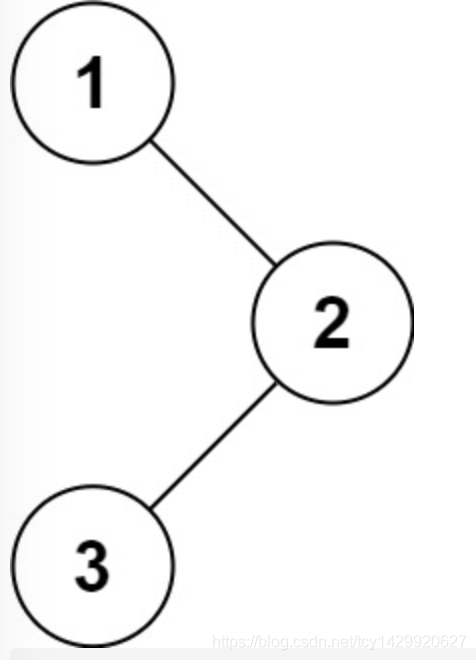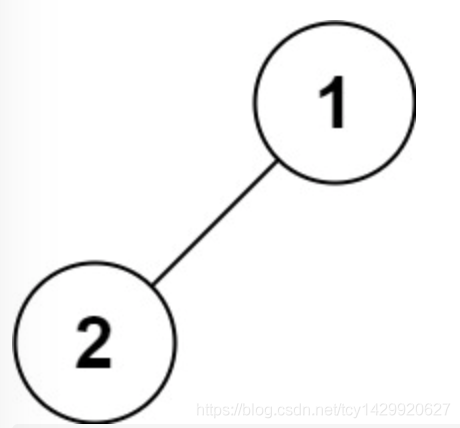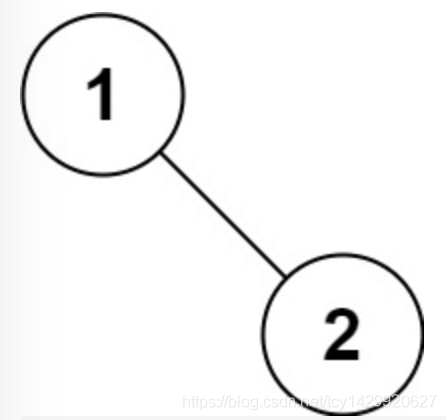# 题目描述-100 <= Node.val <= 100

# 解题代码

``````/**
* Definition for a binary tree node.
* public class TreeNode {
*     int val;
*     TreeNode left;
*     TreeNode right;
*     TreeNode() {}
*     TreeNode(int val) { this.val = val; }
*     TreeNode(int val, TreeNode left, TreeNode right) {
*         this.val = val;
*         this.left = left;
*         this.right = right;
*     }
* }
*/
class Solution {
List<Integer> res = new ArrayList<>();
public List<Integer> preorderTraversal(TreeNode root) {
preorder(root);
return res;
}
public void preorder(TreeNode root) {
if(root == null) {
return;
}